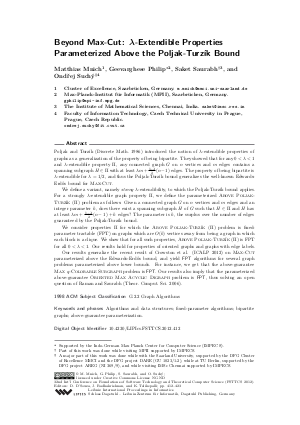Document# Beyond Max-Cut: lambda-Extendible Properties Parameterized Above the Poljak-Turzik Bound

### Authors Matthias Mnich, Geevarghese Philip, Saket Saurabh, Ondrej Suchy## File

LIPIcs.FSTTCS.2012.412.pdf
• Filesize: 0.58 MB
• 12 pages

## Cite As

Matthias Mnich, Geevarghese Philip, Saket Saurabh, and Ondrej Suchy. Beyond Max-Cut: lambda-Extendible Properties Parameterized Above the Poljak-Turzik Bound. In IARCS Annual Conference on Foundations of Software Technology and Theoretical Computer Science (FSTTCS 2012). Leibniz International Proceedings in Informatics (LIPIcs), Volume 18, pp. 412-423, Schloss Dagstuhl - Leibniz-Zentrum für Informatik (2012)
https://doi.org/10.4230/LIPIcs.FSTTCS.2012.412

## Abstract

Poljak and Turzík (Discrete Math. 1986) introduced the notion of lambda-extendible properties of graphs as a generalization of the property of being bipartite. They showed that for any 0 < lambda < 1 and lambda-extendible property Pi, any connected graph G on n vertices and m edges contains a spanning subgraph H in Pi with at least lambda m+ (1-lambda)/2 (n-1) edges. The property of being bipartite is lambda-extendible for lambda=1/2, and thus the Poljak-Turzík bound generalizes the well-known Edwards-Erdos bound for MAXCUT. We define a variant, namely strong lambda-extendibility, to which the Poljak-Turzík bound applies. For a strong lambda-extendible graph property \Pi, we define the parameterized Above Poljak-Turzík problem as follows: Given a connected graph G on n vertices and m edges and an integer parameter k, does there exist a spanning subgraph H of G such that H in Pi and H has at least lambda m+ (1-lambda)/2 (n-1)+k edges? The parameter is k, the surplus over the number of edges guaranteed by the Poljak-Turzík bound. We consider properties Pi for which the Above Poljak-Turzík problem is fixed-parameter tractable (FPT) on graphs which are O(k) vertices away from being a graph in which each block is a clique. We show that for all such properties, Above Poljak-Turzík is FPT for all 0< lambda <1. Our results hold for properties of oriented graphs and graphs with edge labels. Our results generalize the recent result of Crowston et al. (ICALP 2012) on MAXCUT parameterized above the Edwards-Erdos, and yield FPT algorithms for several graph problems parameterized above lower bounds. For instance, we get that the above-guarantee Max q-Colorable Subgraph problem is FPT. Our results also imply that the parameterized above-guarantee Oriented Max Acyclic Digraph problem thus solving an open question of Raman and Saurabh (Theor. Comput. Sci. 2006).
##### Keywords
• Algorithms and data structures; fixed-parameter algorithms; bipartite graphs; above-guarantee parameterization

## Metrics

• Access Statistics
• Total Accesses (updated on a weekly basis)
0